# EMI Calculator using Salesforce Flow

In this article we provide a sample implementation of an EMI calculator using Salesforce Flows. A screen is built that takes three inputs: Amount of the loan, interest rate, loan tenure in months. The formula used for calculating the EMI isHere E is the EMI, P is the amount, r is the interest rate, and n is the number of installments.In the first screen the application takes three inputs P, r and n. In the second screen the results are printed out.

### Introduction

Automation or the practice of having software and machines perform routine tasks is taking over and is here to stay in almost every industry around us. Salesforce Flows are exactly that, it is an automation tool which provides a declarative process automation for Salesforce app, experience, and portal. Salesforce gives us the functionality through Flow Builder to fully automate a guided visual experience which can be implemented in almost all processes to bring some form of automation. Whether it is a Sales rep using a discount calculator to see the discounts he can offer based on the order amount, or him calculating his commission on that order. Flow Builder can automate that!
Here, we will be implementing a Flow to automatically calculate the EMI based on the required inputs which are:

1: Amount of the loan (P)

2: The rate of interest (r)

3: The tenure of the loan (n)

### Implementation Details

Essentially, we will be creating a two-screen flow here, where the first screen would be responsible for getting the required input variables from the user, such as the amount, interest, and loan tenure. The second screen will display the result.
As here we don’t need to store records. We can carry out the process by entering the input values into the formula field itself. We do not need to create variables to store values. However, that would not be the case if we needed the values produced by the flow to be stored. The flow will require 2 screen elements, 3 input fields, and 1 formula field to execute the required flow. Here is a step by step guide of the following:

• We start by selecting a Screen flow as here we have to automate a business process that collects data from users. We can choose any layout, but freeform layout gives us more room to distribute elements in a way that makes it easier for us to understand.

•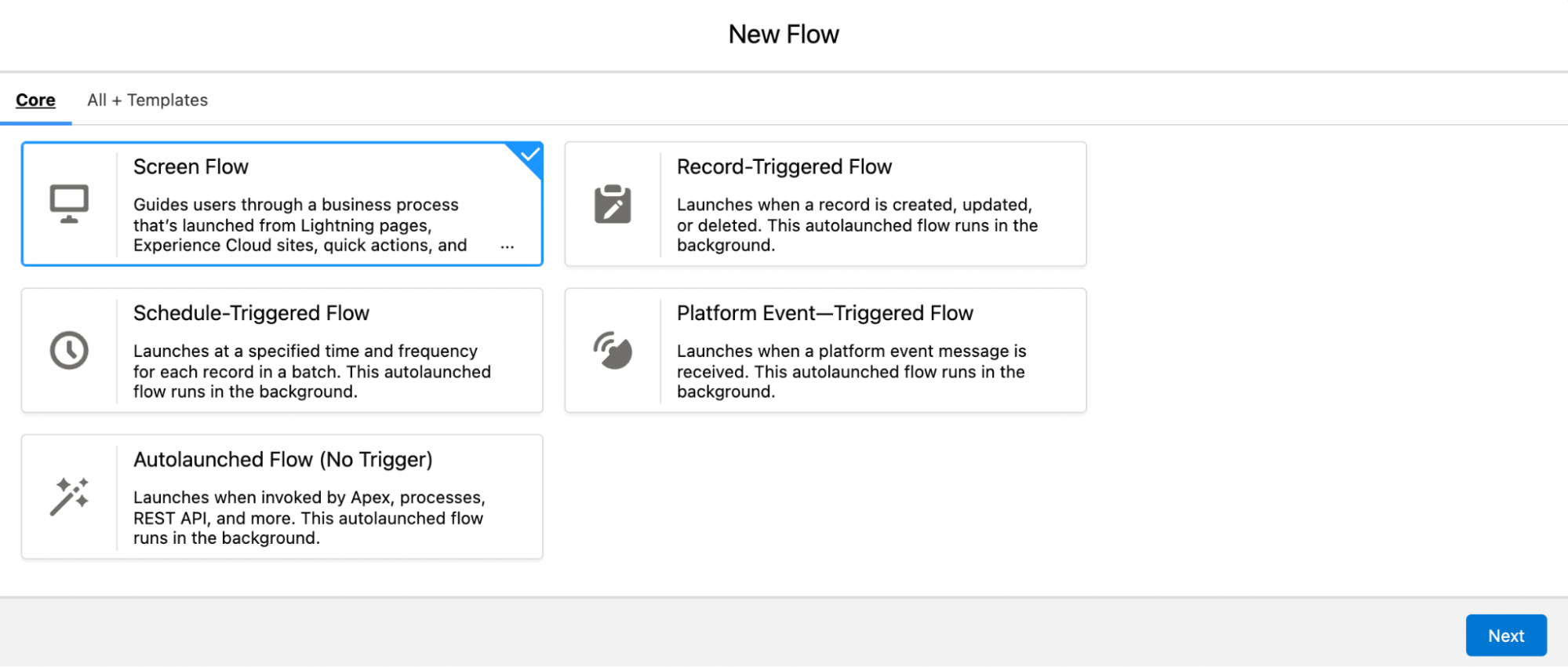• We continue by adding one screen element on the flow and connect the Start to the screen element. In this element we need to add 3 input components, which will be 2 number components for the interest rate (2 decimal places) and loan tenure in months (0 decimal places). The third input component would be of the currency type, which would be the Loan amount.

•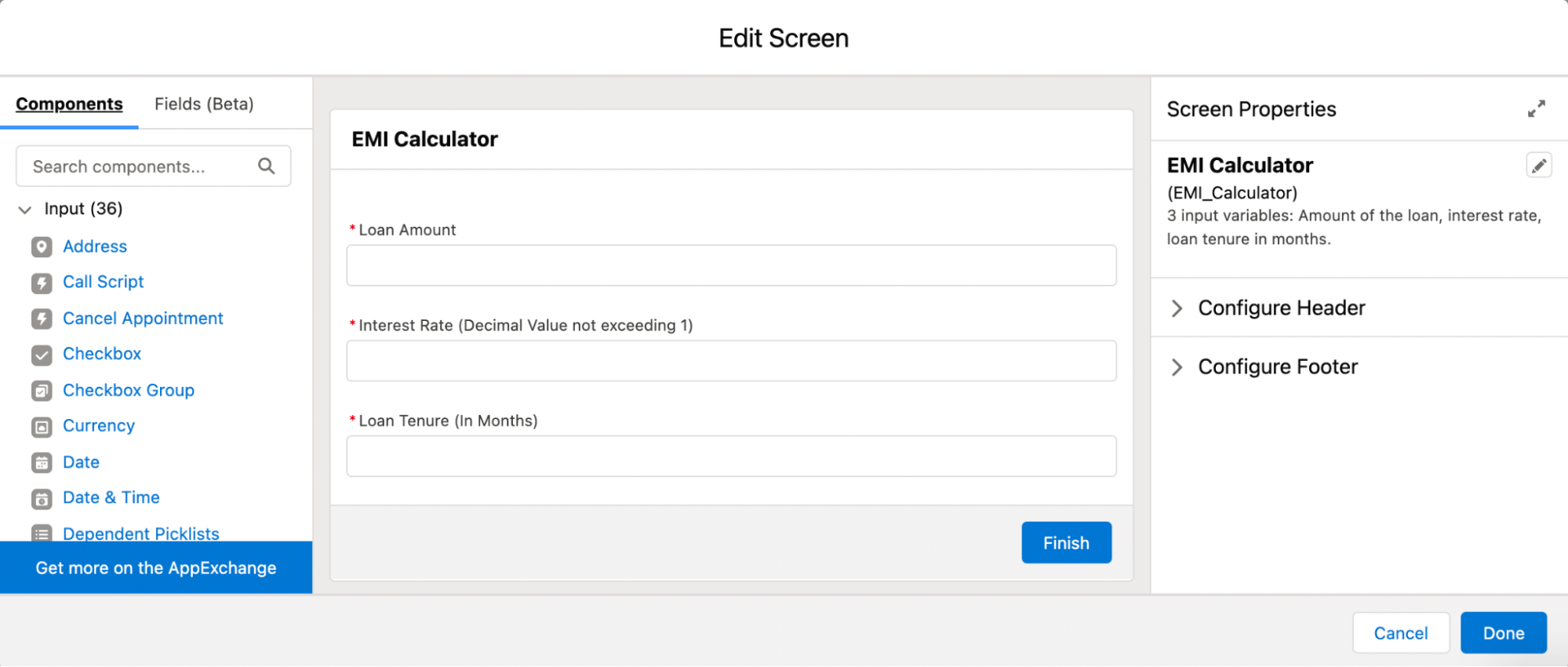• Then we go to the resource manager and get a formula field of the currency data type as it would represent the final loan amount with 2 decimal places, and enter the formula required below.

•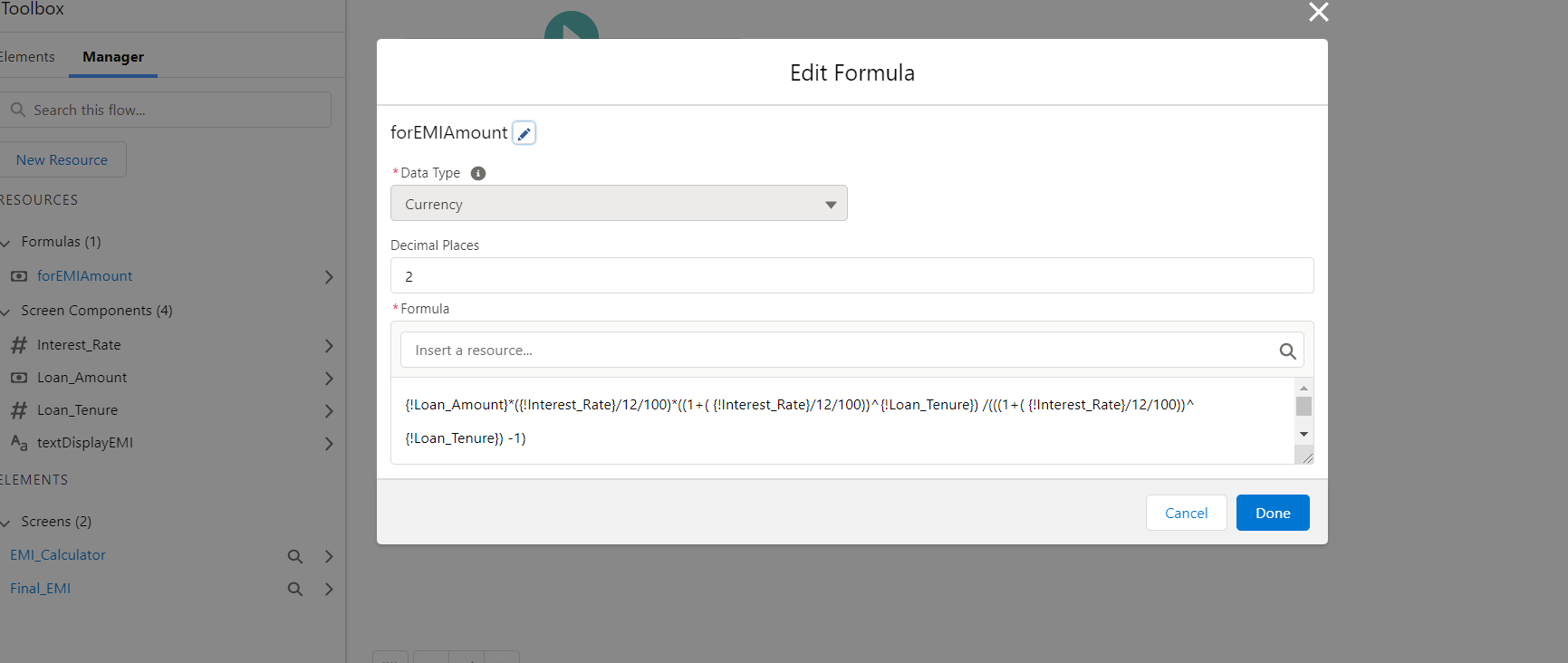• Then we add another screen element which will be used to display the final EMI calculated. Only one display text component is required as we do not take any input here. The display text will output the final value of the formula field as given below in the image.

•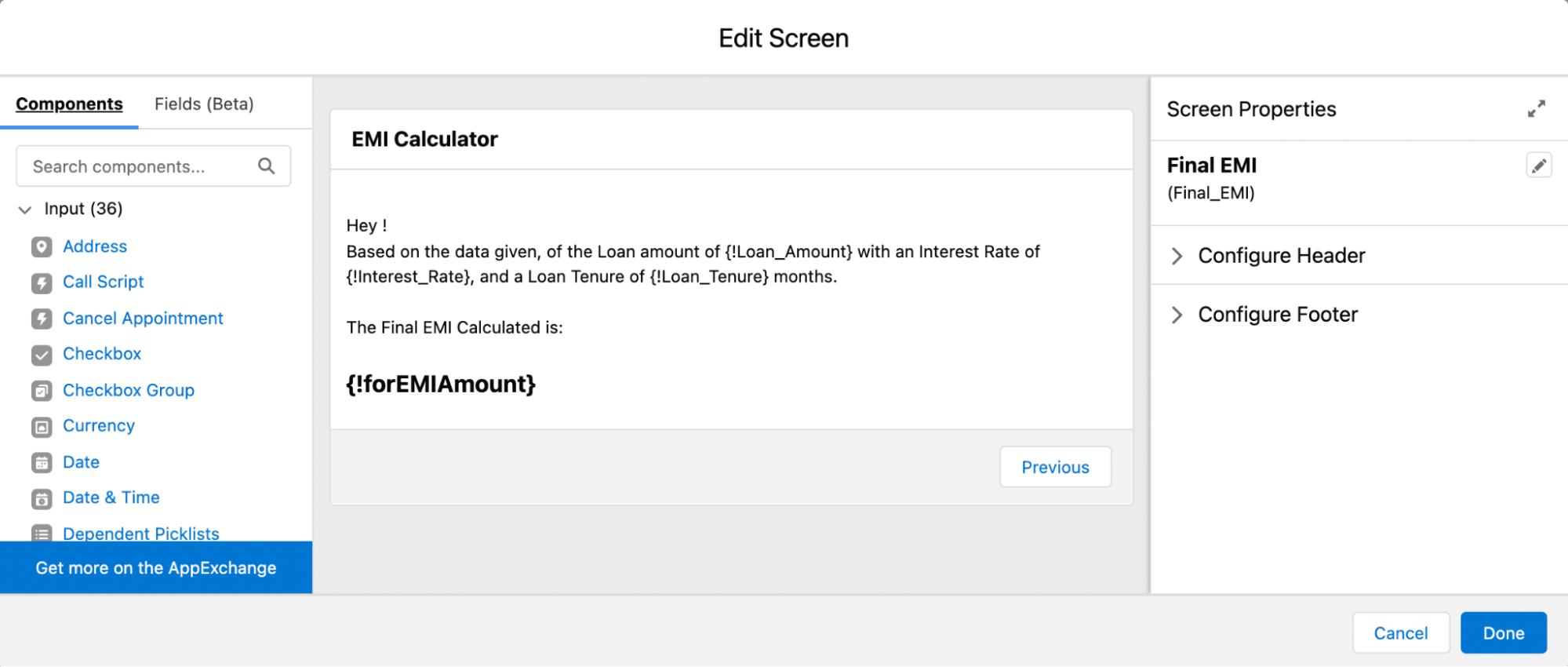Connect the first screen to the second and save the process to have an EMI calculator using Salesforce Flows!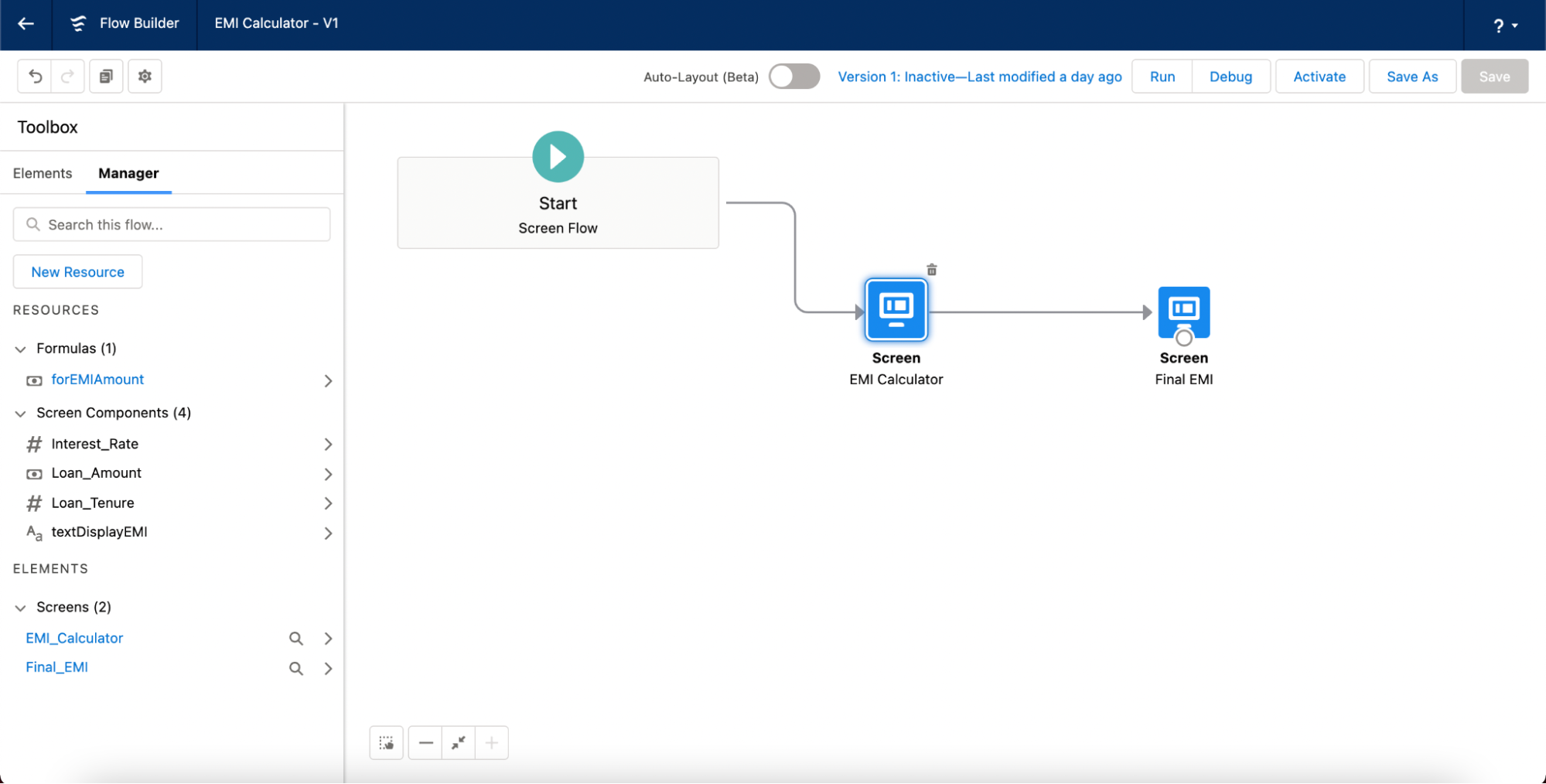### Example:

In the first screen we will enter the loan amount, interest rate and the tenure of the loan . after that we click on next button to see the result.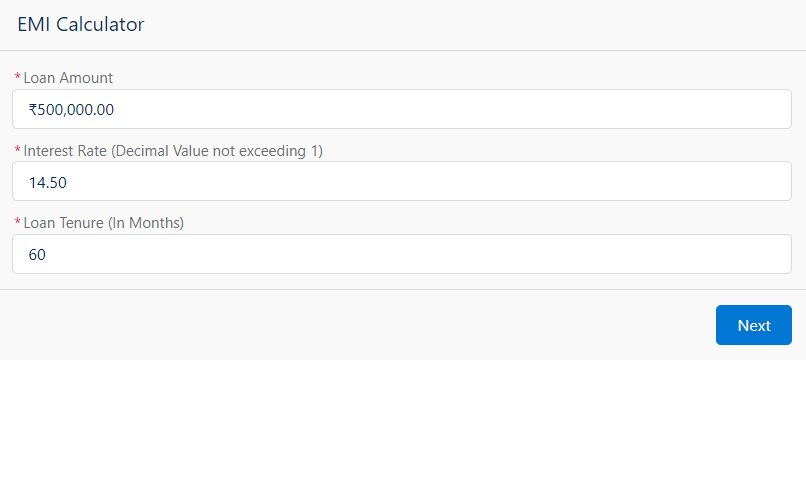In the next screen we will see the final EMI calculation as per the inputs.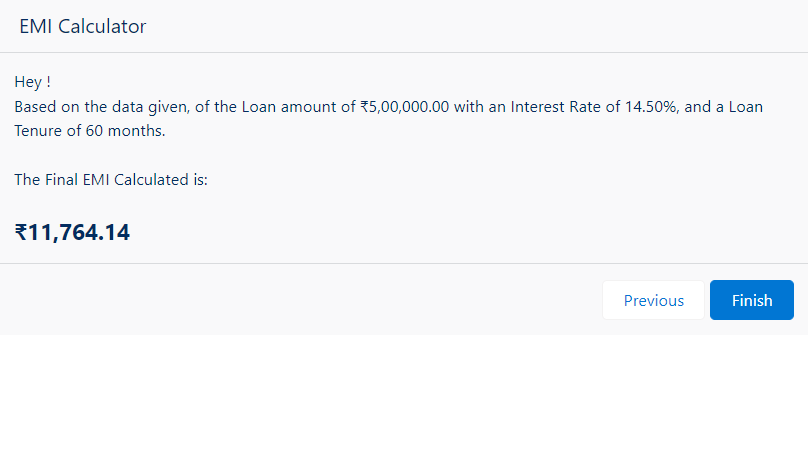### Summary

Screen based flows are extremely useful in automating processes where Screens are needed to take inputs from the user and display the required information. It allows us to integrate various systems into one user-friendly interface. The main benefit is that it gives users the ability to configure process logic and be in full control of the design of the end- user experience without an extensive knowledge of Apex and programming principles.